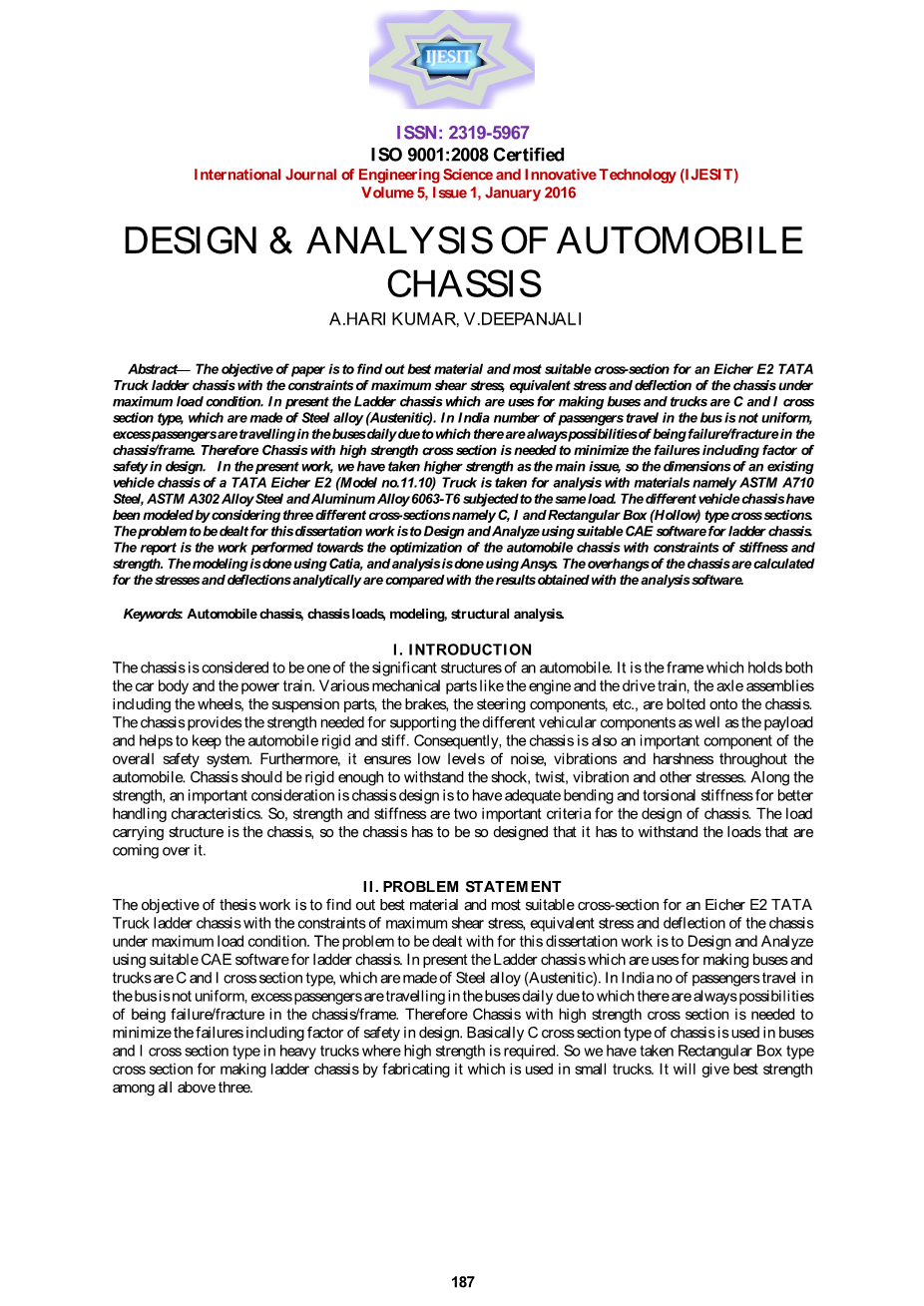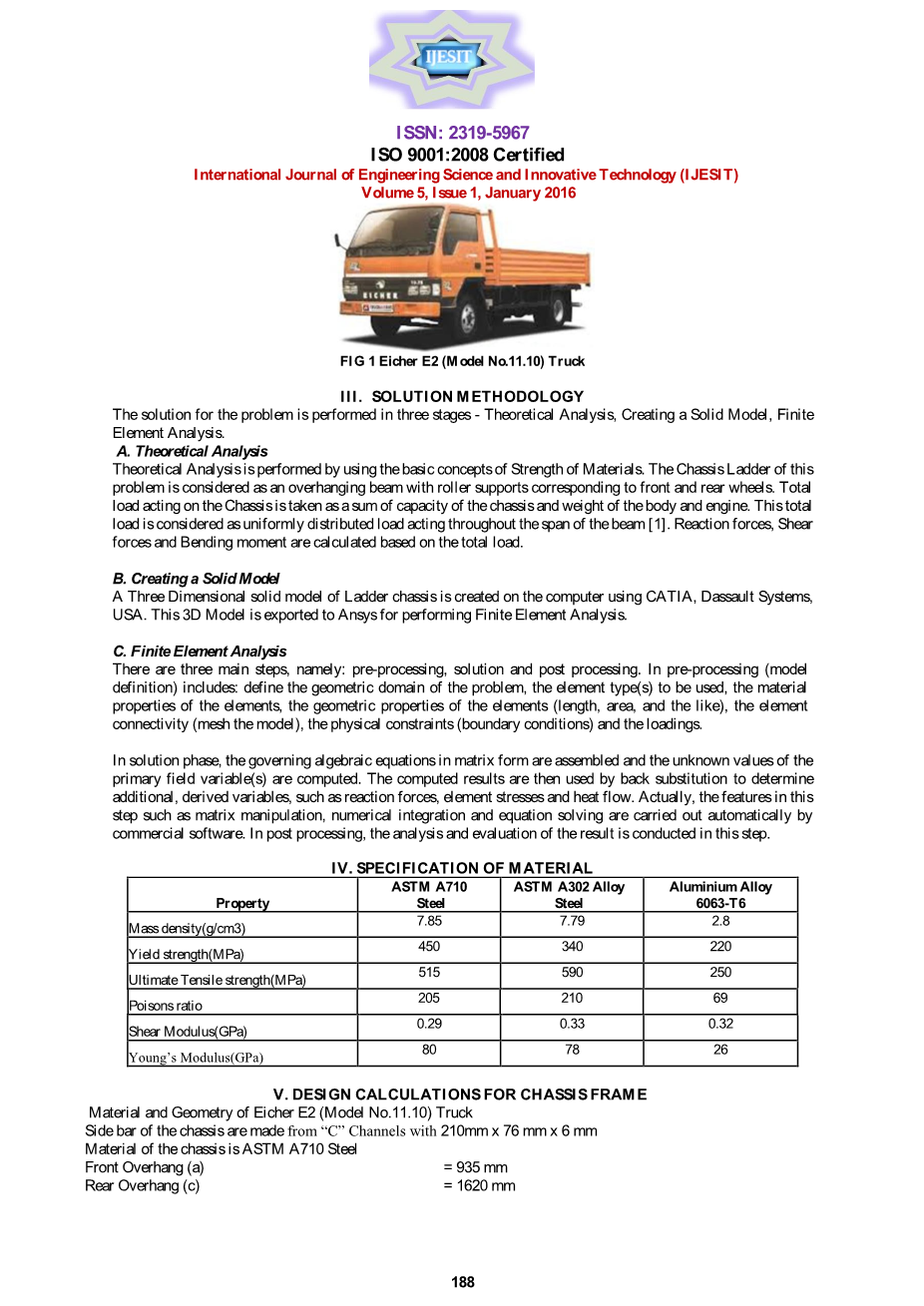# 汽车底盘设计与分析外文翻译资料

2021-11-05 09:11# ISO9001：2008认证

A.HARI KUMAR，V.DEEPANJALI

Ⅰ.导言

II．问题陈述

# ISSN：2319-5967 ISO 9001：2008认证

III．解决方法

A.理论分析

B.创建实体模型

C.有限元分析

IV．材料规格

ASTM A710 ASTM A302合金

7.85 7.79

450 340

6063-T6

2.8

220

515

205

0.29

80

590

210

0.33

78

250

69

0.32

26

V.底盘框架的设计计算Eicher E2（型号11.10）卡车的材料和几何形状

188

# ISSN：2319-5967 ISO 9001：2008认证

= 3800毫米

5 2

= 2.10times;10

N / mm

= 98100 19620

= 117720 N.

= 58860 N / Beam

A.反应计算

= 9.26N / mm 为了获得反应C 和D 的负荷，考虑C 的时刻，我们得到反应负荷

9.26times;935times;2分之935

R

d用在梁上的总载荷

=（9.26times;3800times;3800/2） - （R

= 34727.65 N.

= 9.26times;6355

= 58847.3 N.

times;3800） （9.26times;162

d

R R

R c d

c

= 58847.3

= 24119.65 N.

B.剪切力和弯矩的计算：

F

F a

c

F

d

F

= 0N

=（-9.26times;935） 24119.65

= 15461.55 N.

=（ - 9.16times;4735） 34727.65 24119.65

= 15001.2 N.

= 0N

M

M a

c

M

d

M

b

= 0 Nmm

=（-9.26times;935times;935）/ 2

= -4047661.75 Nmm

= [（-9.26times;4735times;4735）/ 2] （24119.65times;3800）

= -12150971.75 Nmm

= 0 Nmm

189

ISSN：2319-5967 ISO 9001：2008认证

C.弯曲应力计算

4

I = 13372380毫米

= 12150971.75 Nmm

max

2

= 95 N / mm

D.剪切应力计算

theta; = 1°times;

，1,2 =

= 3800毫米

2

= 78.125N / mm

==

=（5737971.47times;2250）/ 26744760

2

= 482.72N / mm

=

= 448.5 MPa

1

2 最大剪切应力

=

190

ISSN：2319-5967 ISO 9001：2008认证

= 257.5 MPa

=

W

X

=机箱重量

= 58860 N.

a，b和c分别是前悬，轮座和后悬。

=总长度/ 2

= 2.66毫米

VI．用CATIA设计底盘框架

C 型横截面梯形底盘的三维模型，I 型横截面和矩形盒型横截面分别如图3,4和5所示。

Ⅶ.用ANSYS工作台进行混沌的有限元分析

1. 网格划分和边界条件

191

ISSN：2319-5967 ISO 9001：2008认证

1. 梯形底盘的结构分析

C型横截面型梯形底盘的结构分析

1. 矩形箱横截面型梯形底盘的结构分析